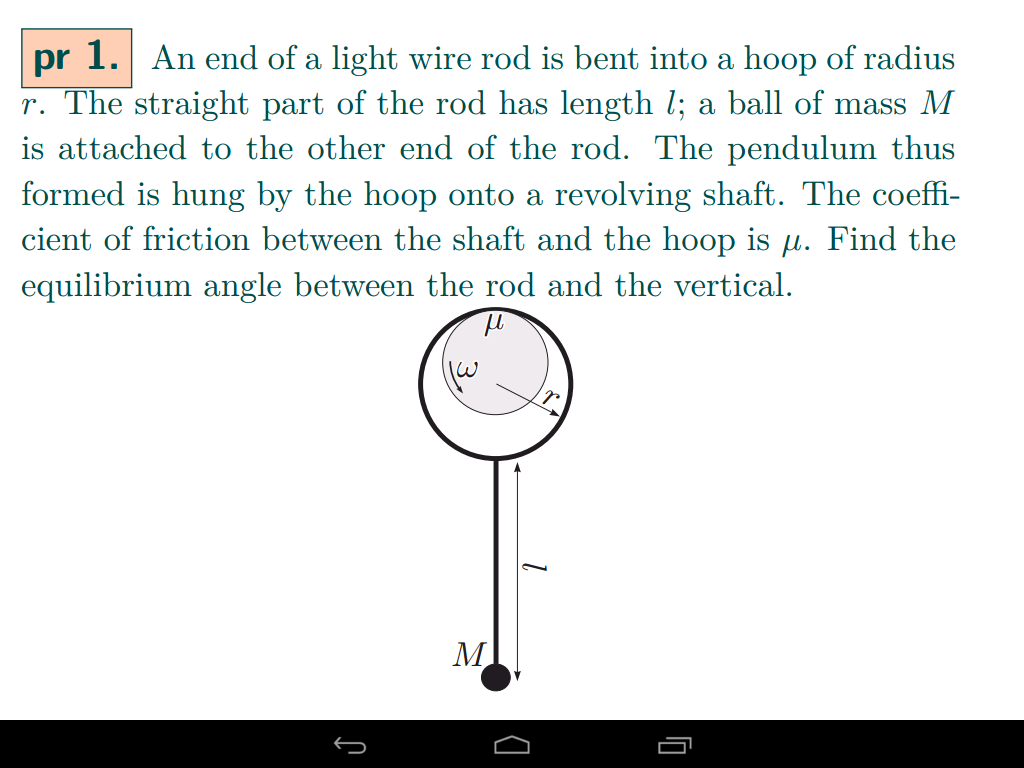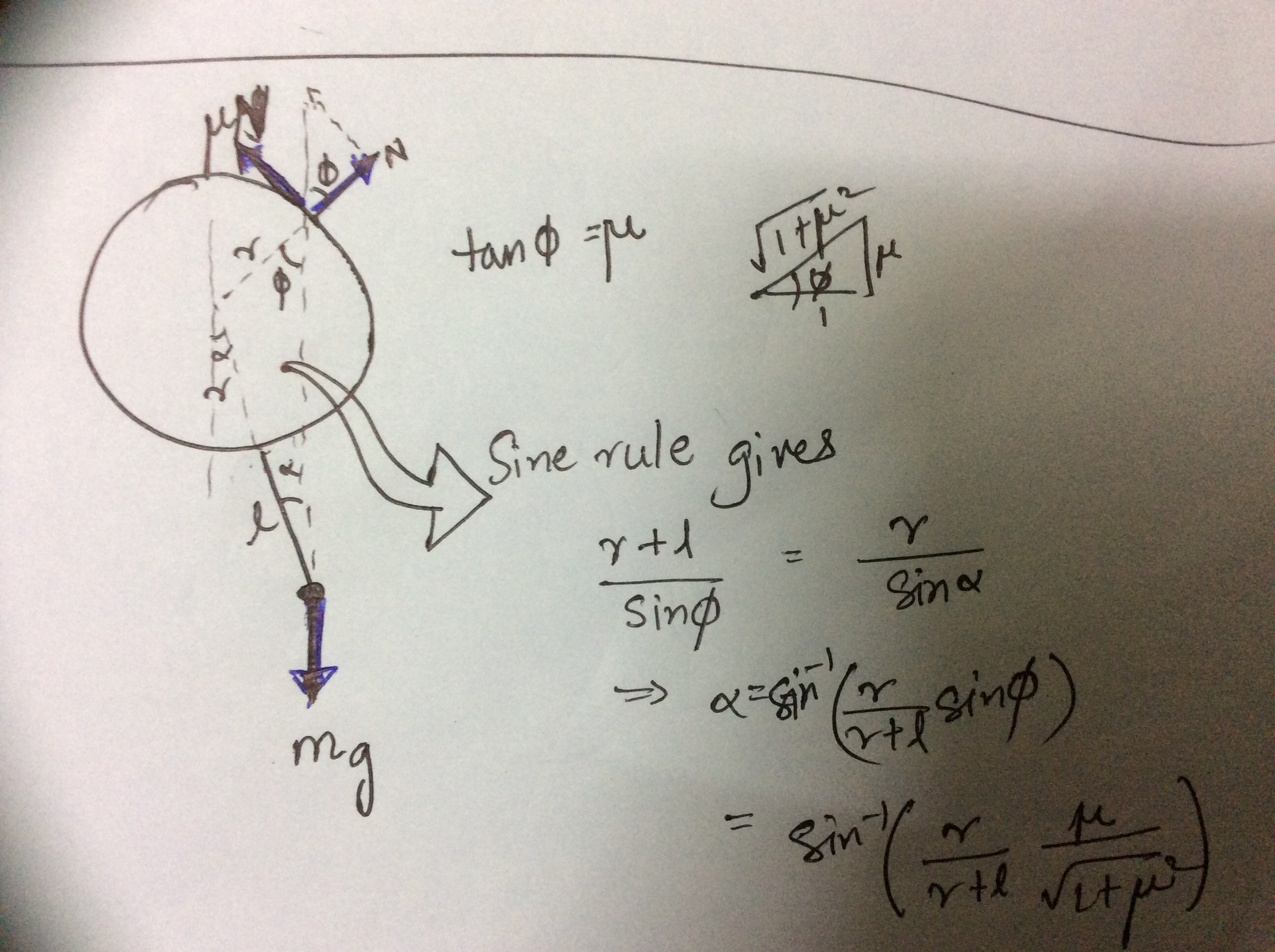# A doubtNote by A Former Brilliant Member
3 years, 4 months ago

This discussion board is a place to discuss our Daily Challenges and the math and science related to those challenges. Explanations are more than just a solution — they should explain the steps and thinking strategies that you used to obtain the solution. Comments should further the discussion of math and science.

When posting on Brilliant:

• Use the emojis to react to an explanation, whether you're congratulating a job well done , or just really confused .
• Ask specific questions about the challenge or the steps in somebody's explanation. Well-posed questions can add a lot to the discussion, but posting "I don't understand!" doesn't help anyone.
• Try to contribute something new to the discussion, whether it is an extension, generalization or other idea related to the challenge.

MarkdownAppears as
*italics* or _italics_ italics
**bold** or __bold__ bold
- bulleted- list
• bulleted
• list
1. numbered2. list
1. numbered
2. list
Note: you must add a full line of space before and after lists for them to show up correctly
paragraph 1paragraph 2

paragraph 1

paragraph 2

[example link](https://brilliant.org)example link
> This is a quote
This is a quote
    # I indented these lines
# 4 spaces, and now they show
# up as a code block.

print "hello world"
# I indented these lines
# 4 spaces, and now they show
# up as a code block.

print "hello world"
MathAppears as
Remember to wrap math in $$ ... $$ or $ ... $ to ensure proper formatting.
2 \times 3 $2 \times 3$
2^{34} $2^{34}$
a_{i-1} $a_{i-1}$
\frac{2}{3} $\frac{2}{3}$
\sqrt{2} $\sqrt{2}$
\sum_{i=1}^3 $\sum_{i=1}^3$
\sin \theta $\sin \theta$
\boxed{123} $\boxed{123}$

Sort by:- 3 years ago

Thanks!!!

- 3 years, 4 months ago

- 3 years, 4 months ago

Thanks for the q.let me try.

- 3 years, 4 months ago

Has anybody solved this yet?

- 3 years, 3 months ago

Any ideas guyzzz????

- 3 years, 3 months ago

Tan(Equlibruim angle)= (ur)/(l+r) Is that right ??

- 3 years, 3 months ago

Torque applies here??

- 3 years, 2 months ago

Neat. I like this. I have to go now, but will come back and check this.

The way to approach this is to think equilibrium. If the thin rod is not moving, then it is in equilibrium. All torques (taken about any convenient point must sum to zero. And all forces must sum to zero.

Let mu the coefficient of friction be u... So imagine that u is large and the rod is horizontal. The CCW rotating axle gives the hoop a leftward pointing force. Take torques about the center of the hoop. That force is mu* m * g and it makes a torque out of the page equal to

r * u * m * g The bob makes a torque = m * g * (l+r) sine (theta) into the page and that is equal to the first torque.

So sine (theta) = r * u * m * g / ( m * g * ( l+r) ) = r * u / ( l+r)

Thus theta = arcsine ( r * u / ( l+r ) ) This is true for the hoop touching the axle at the top of the hoop.

it will touch at an angle though, and that will make the mg break into components, in the r * u * m * g part. So that is messier. I will come back to this.

- 3 years, 1 month ago

I'm facing a problem regarding which books to refer, A Das Gupta or Cengage (As subjective might make me slow and objective might not ensure conceptual clarity). Could you kindly comment?

- 2 years, 11 months ago

i actually use cengage for both physics and maths. Cengage has quite a good number of subjective questions in each chapter,so you should not worry about conceptual clarity.

- 2 years, 11 months ago

Thanks!

- 2 years, 11 months ago